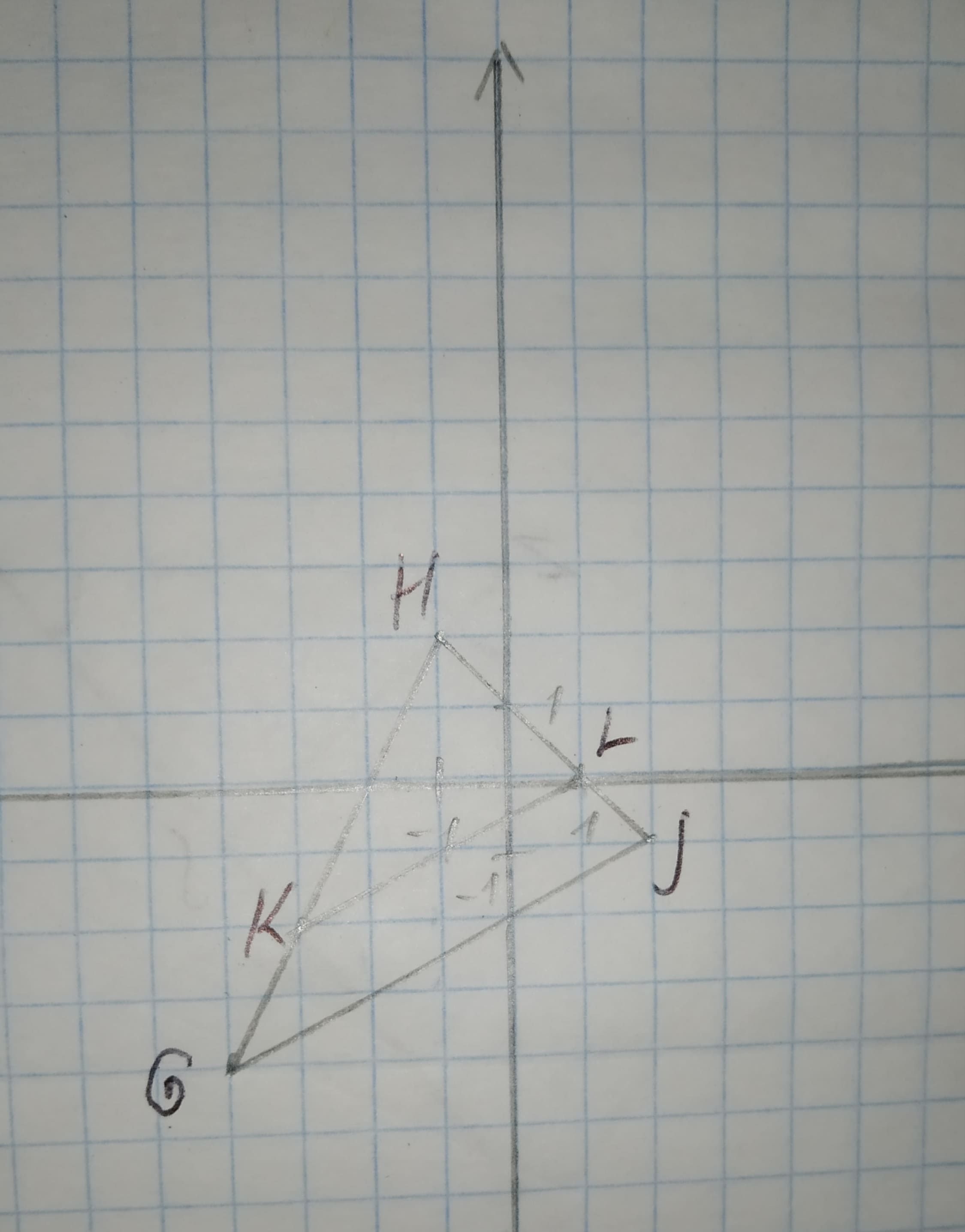# To sketch:The graph from the points and verify that the dilation is a similarity transformation. Given information: G(-4,-4),H(-1,2),J(2,-1),K(-3,-2)L(1,0).CoormaBak9 2020-12-30 Answered
To sketch:The graph from the points and verify that the dilation is a similarity transformation.
Given information:
G(-4,-4),H(-1,2),J(2,-1),K(-3,-2)L(1,0).
You can still ask an expert for help

• Questions are typically answered in as fast as 30 minutes

Solve your problem for the price of one coffee

• Math expert for every subject
• Pay only if we can solve itNathanael Webber

Graph:
Consider the given points G(-4,-4),H(-1,2),J(2,-1),K(-3,-2)L(1,0).
Now, draw the graph.Interpretation:
From the figure there are two triangles $\mathrm{△}HKL\phantom{\rule{1em}{0ex}}\text{and}\phantom{\rule{1em}{0ex}}\mathrm{△}HGJ$.
Now,
Slop of KL = Slop of $CJ=\frac{1}{2}$
Thus, $KL\mid \mid GJ$
Thus, the corresponding angles are congruent.
Thus, by AA similarity theorem triangles are similar.